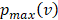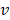#### Contact Mechanics for a Ball Dropped on an Infinite Plate, an Update

##### Publisher: IEOM Society International
0 Paper Citations
1 Views
##### Track: Mathematical Sciences

Contact mechanics has been largely used for the prediction of the behavior of material internal stresses due to external interactions. The analysis of these problems in the elastic regime was first done by Hertz, who focused on the behavior of the stresses in the near vicinities of the contact. The case of a ball interacting with an infinite plate is a special case of Hertz’s theory, which was originally designed for the interaction between two spheres. For this case, classical equations for the prediction of maximum normal pressure and maximum reaction force are still being used, which date as back as 1948. These equations however, seem to significantly overestimate the maximum normal pressure generated by the interactions of these two bodies, an observation also induced by the authors which first derived these results. Besides the presence of questionable theoretical basis, a significant proportion of the literature still takes these models as the only and irrefutable source of theoretical estimations. This paper shows the derivation of new contact mechanics equations for the case of a ball being dropped on an infinite plate of the same material. The derivation of these equations takes Hertz’s theory as a fundamental preliminary. It assumes a sphere of radius R falling from a vertical distance h from the plate. The contact is assumed to be elastic, and so the Work-Energy Theorem can be used to calculate the work done by the plate on the sphere. Given that the integral solution for the work experienced by the sphere using this method is complex, a series expansion around the origin is used. Additionally, given that the change in the independent variable is significantly small, the first term of this expansion results in a good approximation of the original solution. Therefore, the proposed model is:

pmaxv=ER2-R-51.51-?2??R2.5v232E0.420.5R1-?2?Where pmaxvis the maximum normal stress experienced, E is the Young’s modulus, ?is the Poisson’s ratio, vthe speed of the ball right before contacting the plate and ?the material’s density. This model, compared to the classical equations, takes into account the ball radius and predicts much lower maximum normal pressures by predicting a lager contact area, as expected.

Published in: 1st Australian International Conference on Industrial Engineering and Operations Management, Sydney, Australia

Publisher: IEOM Society International
Date of Conference: December 21-22, 2022

ISBN: 979-8-3507-0542-3
ISSN/E-ISSN: 2169-8767